Elementary Algebra 2e

# 6.3Multiply Polynomials

Elementary Algebra 2e6.3 Multiply Polynomials

### Learning Objectives

By the end of this section, you will be able to:

• Multiply a polynomial by a monomial
• Multiply a binomial by a binomial
• Multiply a trinomial by a binomial

### Be Prepared 6.6

Before you get started, take this readiness quiz.

Distribute: $2(x+3).2(x+3).$
If you missed this problem, review Example 1.132.

### Be Prepared 6.7

Combine like terms: $x2+9x+7x+63.x2+9x+7x+63.$
If you missed this problem, review Example 1.24.

### Multiply a Polynomial by a Monomial

We have used the Distributive Property to simplify expressions like $2(x−3)2(x−3)$. You multiplied both terms in the parentheses, $xand3xand3$, by 2, to get $2x−62x−6$. With this chapter’s new vocabulary, you can say you were multiplying a binomial, $x−3x−3$, by a monomial, 2.

Multiplying a binomial by a monomial is nothing new for you! Here’s an example:

### Example 6.28

Multiply: $4(x+3).4(x+3).$

### Try It 6.55

Multiply: $5(x+7).5(x+7).$

### Try It 6.56

Multiply: $3(y+13).3(y+13).$

### Example 6.29

Multiply: $y(y−2).y(y−2).$

### Try It 6.57

Multiply: $x(x−7).x(x−7).$

### Try It 6.58

Multiply: $d(d−11).d(d−11).$

### Example 6.30

Multiply: $7x(2x+y).7x(2x+y).$

### Try It 6.59

Multiply: $5x(x+4y).5x(x+4y).$

### Try It 6.60

Multiply: $2p(6p+r).2p(6p+r).$

### Example 6.31

Multiply: $−2y(4y2+3y−5).−2y(4y2+3y−5).$

### Try It 6.61

Multiply: $−3y(5y2+8y−7).−3y(5y2+8y−7).$

### Try It 6.62

Multiply: $4x2(2x2−3x+5).4x2(2x2−3x+5).$

### Example 6.32

Multiply: $2x3(x2−8x+1).2x3(x2−8x+1).$

### Try It 6.63

Multiply: $4x(3x2−5x+3).4x(3x2−5x+3).$

### Try It 6.64

Multiply: $−6a3(3a2−2a+6).−6a3(3a2−2a+6).$

### Example 6.33

Multiply: $(x+3)p.(x+3)p.$

### Try It 6.65

Multiply: $(x+8)p.(x+8)p.$

### Try It 6.66

Multiply: $(a+4)p.(a+4)p.$

### Multiply a Binomial by a Binomial

Just like there are different ways to represent multiplication of numbers, there are several methods that can be used to multiply a binomial times a binomial. We will start by using the Distributive Property.

#### Multiply a Binomial by a Binomial Using the Distributive Property

Look at Example 6.33, where we multiplied a binomial by a monomial.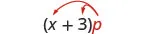We distributed the p to get: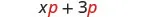What if we have (x + 7) instead of p?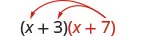Distribute (x + 7).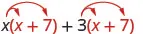Distribute again.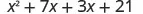Combine like terms.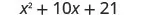Notice that before combining like terms, you had four terms. You multiplied the two terms of the first binomial by the two terms of the second binomial—four multiplications.

### Example 6.34

Multiply: $(y+5)(y+8).(y+5)(y+8).$

### Try It 6.67

Multiply: $(x+8)(x+9).(x+8)(x+9).$

### Try It 6.68

Multiply: $(5x+9)(4x+3).(5x+9)(4x+3).$

### Example 6.35

Multiply: $(2y+5)(3y+4).(2y+5)(3y+4).$

### Try It 6.69

Multiply: $(3b+5)(4b+6).(3b+5)(4b+6).$

### Try It 6.70

Multiply: $(a+10)(a+7).(a+10)(a+7).$

### Example 6.36

Multiply: $(4y+3)(2y−5).(4y+3)(2y−5).$

### Try It 6.71

Multiply: $(5y+2)(6y−3).(5y+2)(6y−3).$

### Try It 6.72

Multiply: $(3c+4)(5c−2).(3c+4)(5c−2).$

### Example 6.37

Multiply: $(x-2)(x−y).(x-2)(x−y).$

### Try It 6.73

Multiply: $(a+7)(a−b).(a+7)(a−b).$

### Try It 6.74

Multiply: $(x+5)(x−y).(x+5)(x−y).$

#### Multiply a Binomial by a Binomial Using the FOIL Method

Remember that when you multiply a binomial by a binomial you get four terms. Sometimes you can combine like terms to get a trinomial, but sometimes, like in Example 6.37, there are no like terms to combine.

Let’s look at the last example again and pay particular attention to how we got the four terms.

$(x−2)(x−y)x2−xy−2x+2y(x−2)(x−y)x2−xy−2x+2y$

Where did the first term, $x2x2$, come from?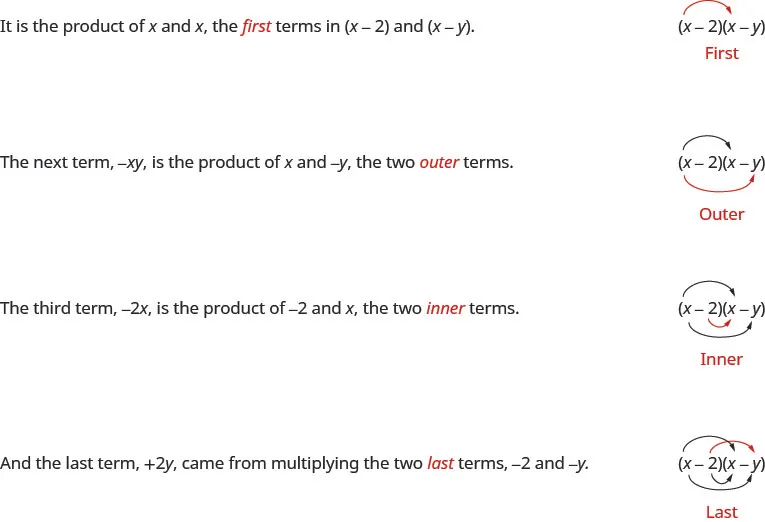We abbreviate “First, Outer, Inner, Last” as FOIL. The letters stand for ‘First, Outer, Inner, Last’. The word FOIL is easy to remember and ensures we find all four products.

$(x−2)(x−y)x2−xy−2x+2yFOIL(x−2)(x−y)x2−xy−2x+2yFOIL$

Let’s look at $(x+3)(x+7)(x+3)(x+7)$.

 Distibutive Property FOIL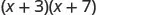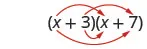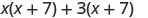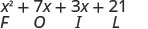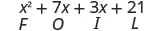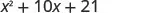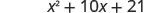Notice how the terms in third line fit the FOIL pattern.

Now we will do an example where we use the FOIL pattern to multiply two binomials.

### Example 6.38

#### How to Multiply a Binomial by a Binomial using the FOIL Method

Multiply using the FOIL method: $(x+5)(x+9).(x+5)(x+9).$

### Try It 6.75

Multiply using the FOIL method: $(x+6)(x+8).(x+6)(x+8).$

### Try It 6.76

Multiply using the FOIL method: $(y+17)(y+3).(y+17)(y+3).$

We summarize the steps of the FOIL method below. The FOIL method only applies to multiplying binomials, not other polynomials!

### How To

#### Multiply two binomials using the FOIL method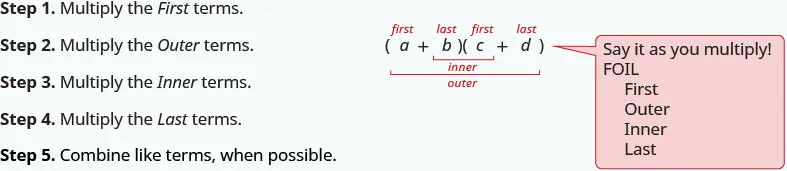When you multiply by the FOIL method, drawing the lines will help your brain focus on the pattern and make it easier to apply.

### Example 6.39

Multiply: $(y−7)(y+4).(y−7)(y+4).$

### Try It 6.77

Multiply: $(x−7)(x+5).(x−7)(x+5).$

### Try It 6.78

Multiply: $(b−3)(b+6).(b−3)(b+6).$

### Example 6.40

Multiply: $(4x+3)(2x−5).(4x+3)(2x−5).$

### Try It 6.79

Multiply: $(3x+7)(5x−2).(3x+7)(5x−2).$

### Try It 6.80

Multiply: $(4y+5)(4y−10).(4y+5)(4y−10).$

The final products in the last four examples were trinomials because we could combine the two middle terms. This is not always the case.

### Example 6.41

Multiply: $(3x−y)(2x−5).(3x−y)(2x−5).$

### Try It 6.81

Multiply: $(10c−d)(c−6).(10c−d)(c−6).$

### Try It 6.82

Multiply: $(7x−y)(2x−5).(7x−y)(2x−5).$

Be careful of the exponents in the next example.

### Example 6.42

Multiply: $(n2+4)(n−1).(n2+4)(n−1).$

### Try It 6.83

Multiply: $(x2+6)(x−8).(x2+6)(x−8).$

### Try It 6.84

Multiply: $(y2+7)(y−9).(y2+7)(y−9).$

### Example 6.43

Multiply: $(3pq+5)(6pq−11).(3pq+5)(6pq−11).$

### Try It 6.85

Multiply: $(2ab+5)(4ab−4).(2ab+5)(4ab−4).$

### Try It 6.86

Multiply: $(2xy+3)(4xy−5).(2xy+3)(4xy−5).$

#### Multiply a Binomial by a Binomial Using the Vertical Method

The FOIL method is usually the quickest method for multiplying two binomials, but it only works for binomials. You can use the Distributive Property to find the product of any two polynomials. Another method that works for all polynomials is the Vertical Method. It is very much like the method you use to multiply whole numbers. Look carefully at this example of multiplying two-digit numbers.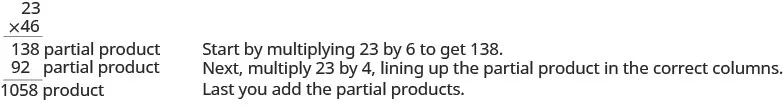Now we’ll apply this same method to multiply two binomials.

### Example 6.44

Multiply using the Vertical Method: $(3y−1)(2y−6).(3y−1)(2y−6).$

### Try It 6.87

Multiply using the Vertical Method: $(5m−7)(3m−6).(5m−7)(3m−6).$

### Try It 6.88

Multiply using the Vertical Method: $(6b−5)(7b−3).(6b−5)(7b−3).$

We have now used three methods for multiplying binomials. Be sure to practice each method, and try to decide which one you prefer. The methods are listed here all together, to help you remember them.

### Multiplying Two Binomials

To multiply binomials, use the:

• Distributive Property
• FOIL Method
• Vertical Method

Remember, FOIL only works when multiplying two binomials.

### Multiply a Trinomial by a Binomial

We have multiplied monomials by monomials, monomials by polynomials, and binomials by binomials. Now we’re ready to multiply a trinomial by a binomial. Remember, FOIL will not work in this case, but we can use either the Distributive Property or the Vertical Method. We first look at an example using the Distributive Property.

### Example 6.45

Multiply using the Distributive Property: $(b+3)(2b2−5b+8).(b+3)(2b2−5b+8).$

### Try It 6.89

Multiply using the Distributive Property: $(y−3)(y2−5y+2).(y−3)(y2−5y+2).$

### Try It 6.90

Multiply using the Distributive Property: $(x+4)(2x2−3x+5).(x+4)(2x2−3x+5).$

Now let’s do this same multiplication using the Vertical Method.

### Example 6.46

Multiply using the Vertical Method: $(b+3)(2b2−5b+8).(b+3)(2b2−5b+8).$

### Try It 6.91

Multiply using the Vertical Method: $(y−3)(y2−5y+2).(y−3)(y2−5y+2).$

### Try It 6.92

Multiply using the Vertical Method: $(x+4)(2x2−3x+5).(x+4)(2x2−3x+5).$

We have now seen two methods you can use to multiply a trinomial by a binomial. After you practice each method, you’ll probably find you prefer one way over the other. We list both methods are listed here, for easy reference.

### Multiplying a Trinomial by a Binomial

To multiply a trinomial by a binomial, use the:

• Distributive Property
• Vertical Method

### Media

Access these online resources for additional instruction and practice with multiplying polynomials:

### Section 6.3 Exercises

#### Practice Makes Perfect

Multiply a Polynomial by a Monomial

In the following exercises, multiply.

173.

$4 ( w + 10 ) 4 ( w + 10 )$

174.

$6 ( b + 8 ) 6 ( b + 8 )$

175.

$−3 ( a + 7 ) −3 ( a + 7 )$

176.

$−5 ( p + 9 ) −5 ( p + 9 )$

177.

$2 ( x − 7 ) 2 ( x − 7 )$

178.

$7 ( y − 4 ) 7 ( y − 4 )$

179.

$−3 ( k − 4 ) −3 ( k − 4 )$

180.

$−8 ( j − 5 ) −8 ( j − 5 )$

181.

$q ( q + 5 ) q ( q + 5 )$

182.

$k ( k + 7 ) k ( k + 7 )$

183.

$− b ( b + 9 ) − b ( b + 9 )$

184.

$− y ( y + 3 ) − y ( y + 3 )$

185.

$− x ( x − 10 ) − x ( x − 10 )$

186.

$− p ( p − 15 ) − p ( p − 15 )$

187.

$6 r ( 4 r + s ) 6 r ( 4 r + s )$

188.

$5 c ( 9 c + d ) 5 c ( 9 c + d )$

189.

$12 x ( x − 10 ) 12 x ( x − 10 )$

190.

$9 m ( m − 11 ) 9 m ( m − 11 )$

191.

$−9 a ( 3 a + 5 ) −9 a ( 3 a + 5 )$

192.

$−4 p ( 2 p + 7 ) −4 p ( 2 p + 7 )$

193.

$3 ( p 2 + 10 p + 25 ) 3 ( p 2 + 10 p + 25 )$

194.

$6 ( y 2 + 8 y + 16 ) 6 ( y 2 + 8 y + 16 )$

195.

$−8 x ( x 2 + 2 x − 15 ) −8 x ( x 2 + 2 x − 15 )$

196.

$−5 t ( t 2 + 3 t − 18 ) −5 t ( t 2 + 3 t − 18 )$

197.

$5 q 3 ( q 3 − 2 q + 6 ) 5 q 3 ( q 3 − 2 q + 6 )$

198.

$4 x 3 ( x 4 − 3 x + 7 ) 4 x 3 ( x 4 − 3 x + 7 )$

199.

$−8 y ( y 2 + 2 y − 15 ) −8 y ( y 2 + 2 y − 15 )$

200.

$−5 m ( m 2 + 3 m − 18 ) −5 m ( m 2 + 3 m − 18 )$

201.

$5 q 3 ( q 2 − 2 q + 6 ) 5 q 3 ( q 2 − 2 q + 6 )$

202.

$9 r 3 ( r 2 − 3 r + 5 ) 9 r 3 ( r 2 − 3 r + 5 )$

203.

$−4 z 2 ( 3 z 2 + 12 z − 1 ) −4 z 2 ( 3 z 2 + 12 z − 1 )$

204.

$−3 x 2 ( 7 x 2 + 10 x − 1 ) −3 x 2 ( 7 x 2 + 10 x − 1 )$

205.

$( 2 m − 9 ) m ( 2 m − 9 ) m$

206.

$( 8 j − 1 ) j ( 8 j − 1 ) j$

207.

$( w − 6 ) · 8 ( w − 6 ) · 8$

208.

$( k − 4 ) · 5 ( k − 4 ) · 5$

209.

$4 ( x + 10 ) 4 ( x + 10 )$

210.

$6 ( y + 8 ) 6 ( y + 8 )$

211.

$15 ( r − 24 ) 15 ( r − 24 )$

212.

$12 ( v − 30 ) 12 ( v − 30 )$

213.

$−3 ( m + 11 ) −3 ( m + 11 )$

214.

$−4 ( p + 15 ) −4 ( p + 15 )$

215.

$−8 ( z − 5 ) −8 ( z − 5 )$

216.

$−3 ( x − 9 ) −3 ( x − 9 )$

217.

$u ( u + 5 ) u ( u + 5 )$

218.

$q ( q + 7 ) q ( q + 7 )$

219.

$n ( n 2 − 3 n ) n ( n 2 − 3 n )$

220.

$s ( s 2 − 6 s ) s ( s 2 − 6 s )$

221.

$6 x ( 4 x + y ) 6 x ( 4 x + y )$

222.

$5 a ( 9 a + b ) 5 a ( 9 a + b )$

223.

$5 p ( 11 p − 5 q ) 5 p ( 11 p − 5 q )$

224.

$12 u ( 3 u − 4 v ) 12 u ( 3 u − 4 v )$

225.

$3 ( v 2 + 10 v + 25 ) 3 ( v 2 + 10 v + 25 )$

226.

$6 ( x 2 + 8 x + 16 ) 6 ( x 2 + 8 x + 16 )$

227.

$2 n ( 4 n 2 − 4 n + 1 ) 2 n ( 4 n 2 − 4 n + 1 )$

228.

$3 r ( 2 r 2 − 6 r + 2 ) 3 r ( 2 r 2 − 6 r + 2 )$

229.

$−8 y ( y 2 + 2 y − 15 ) −8 y ( y 2 + 2 y − 15 )$

230.

$−5 m ( m 2 + 3 m − 18 ) −5 m ( m 2 + 3 m − 18 )$

231.

$5 q 3 ( q 2 − 2 q + 6 ) 5 q 3 ( q 2 − 2 q + 6 )$

232.

$9 r 3 ( r 2 − 3 r + 5 ) 9 r 3 ( r 2 − 3 r + 5 )$

233.

$−4 z 2 ( 3 z 2 + 12 z − 1 ) −4 z 2 ( 3 z 2 + 12 z − 1 )$

234.

$−3 x 2 ( 7 x 2 + 10 x − 1 ) −3 x 2 ( 7 x 2 + 10 x − 1 )$

235.

$( 2 y − 9 ) y ( 2 y − 9 ) y$

236.

$( 8 b − 1 ) b ( 8 b − 1 ) b$

Multiply a Binomial by a Binomial

In the following exercises, multiply the following binomials using: the Distributive Property the FOIL method the Vertical Method.

237.

$( w + 5 ) ( w + 7 ) ( w + 5 ) ( w + 7 )$

238.

$( y + 9 ) ( y + 3 ) ( y + 9 ) ( y + 3 )$

239.

$( p + 11 ) ( p − 4 ) ( p + 11 ) ( p − 4 )$

240.

$( q + 4 ) ( q − 8 ) ( q + 4 ) ( q − 8 )$

In the following exercises, multiply the binomials. Use any method.

241.

$( x + 8 ) ( x + 3 ) ( x + 8 ) ( x + 3 )$

242.

$( y + 7 ) ( y + 4 ) ( y + 7 ) ( y + 4 )$

243.

$( y − 6 ) ( y − 2 ) ( y − 6 ) ( y − 2 )$

244.

$( x − 7 ) ( x − 2 ) ( x − 7 ) ( x − 2 )$

245.

$( w − 4 ) ( w + 7 ) ( w − 4 ) ( w + 7 )$

246.

$( q − 5 ) ( q + 8 ) ( q − 5 ) ( q + 8 )$

247.

$( p + 12 ) ( p − 5 ) ( p + 12 ) ( p − 5 )$

248.

$( m + 11 ) ( m − 4 ) ( m + 11 ) ( m − 4 )$

249.

$( 6 p + 5 ) ( p + 1 ) ( 6 p + 5 ) ( p + 1 )$

250.

$( 7 m + 1 ) ( m + 3 ) ( 7 m + 1 ) ( m + 3 )$

251.

$( 2 t − 9 ) ( 10 t + 1 ) ( 2 t − 9 ) ( 10 t + 1 )$

252.

$( 3 r − 8 ) ( 11 r + 1 ) ( 3 r − 8 ) ( 11 r + 1 )$

253.

$( 5 x − y ) ( 3 x − 6 ) ( 5 x − y ) ( 3 x − 6 )$

254.

$( 10 a − b ) ( 3 a − 4 ) ( 10 a − b ) ( 3 a − 4 )$

255.

$( a + b ) ( 2 a + 3 b ) ( a + b ) ( 2 a + 3 b )$

256.

$( r + s ) ( 3 r + 2 s ) ( r + s ) ( 3 r + 2 s )$

257.

$( 4 z − y ) ( z − 6 ) ( 4 z − y ) ( z − 6 )$

258.

$( 5 x − y ) ( x − 4 ) ( 5 x − y ) ( x − 4 )$

259.

$( x 2 + 3 ) ( x + 2 ) ( x 2 + 3 ) ( x + 2 )$

260.

$( y 2 − 4 ) ( y + 3 ) ( y 2 − 4 ) ( y + 3 )$

261.

$( x 2 + 8 ) ( x 2 − 5 ) ( x 2 + 8 ) ( x 2 − 5 )$

262.

$( y 2 − 7 ) ( y 2 − 4 ) ( y 2 − 7 ) ( y 2 − 4 )$

263.

$( 5 a b − 1 ) ( 2 a b + 3 ) ( 5 a b − 1 ) ( 2 a b + 3 )$

264.

$( 2 x y + 3 ) ( 3 x y + 2 ) ( 2 x y + 3 ) ( 3 x y + 2 )$

265.

$( 6 p q − 3 ) ( 4 p q − 5 ) ( 6 p q − 3 ) ( 4 p q − 5 )$

266.

$( 3 r s − 7 ) ( 3 r s − 4 ) ( 3 r s − 7 ) ( 3 r s − 4 )$

Multiply a Trinomial by a Binomial

In the following exercises, multiply using the Distributive Property the Vertical Method.

267.

$( x + 5 ) ( x 2 + 4 x + 3 ) ( x + 5 ) ( x 2 + 4 x + 3 )$

268.

$( u + 4 ) ( u 2 + 3 u + 2 ) ( u + 4 ) ( u 2 + 3 u + 2 )$

269.

$( y + 8 ) ( 4 y 2 + y − 7 ) ( y + 8 ) ( 4 y 2 + y − 7 )$

270.

$( a + 10 ) ( 3 a 2 + a − 5 ) ( a + 10 ) ( 3 a 2 + a − 5 )$

In the following exercises, multiply. Use either method.

271.

$( w − 7 ) ( w 2 − 9 w + 10 ) ( w − 7 ) ( w 2 − 9 w + 10 )$

272.

$( p − 4 ) ( p 2 − 6 p + 9 ) ( p − 4 ) ( p 2 − 6 p + 9 )$

273.

$( 3 q + 1 ) ( q 2 − 4 q − 5 ) ( 3 q + 1 ) ( q 2 − 4 q − 5 )$

274.

$( 6 r + 1 ) ( r 2 − 7 r − 9 ) ( 6 r + 1 ) ( r 2 − 7 r − 9 )$

Mixed Practice

275.

$( 10 y − 6 ) + ( 4 y − 7 ) ( 10 y − 6 ) + ( 4 y − 7 )$

276.

$( 15 p − 4 ) + ( 3 p − 5 ) ( 15 p − 4 ) + ( 3 p − 5 )$

277.

$( x 2 − 4 x − 34 ) − ( x 2 + 7 x − 6 ) ( x 2 − 4 x − 34 ) − ( x 2 + 7 x − 6 )$

278.

$( j 2 − 8 j − 27 ) − ( j 2 + 2 j − 12 ) ( j 2 − 8 j − 27 ) − ( j 2 + 2 j − 12 )$

279.

$5 q ( 3 q 2 − 6 q + 11 ) 5 q ( 3 q 2 − 6 q + 11 )$

280.

$8 t ( 2 t 2 − 5 t + 6 ) 8 t ( 2 t 2 − 5 t + 6 )$

281.

$( s − 7 ) ( s + 9 ) ( s − 7 ) ( s + 9 )$

282.

$( x − 5 ) ( x + 13 ) ( x − 5 ) ( x + 13 )$

283.

$( y 2 − 2 y ) ( y + 1 ) ( y 2 − 2 y ) ( y + 1 )$

284.

$( a 2 − 3 a ) ( 4 a + 5 ) ( a 2 − 3 a ) ( 4 a + 5 )$

285.

$( 3 n − 4 ) ( n 2 + n − 7 ) ( 3 n − 4 ) ( n 2 + n − 7 )$

286.

$( 6 k − 1 ) ( k 2 + 2 k − 4 ) ( 6 k − 1 ) ( k 2 + 2 k − 4 )$

287.

$( 7 p + 10 ) ( 7 p − 10 ) ( 7 p + 10 ) ( 7 p − 10 )$

288.

$( 3 y + 8 ) ( 3 y − 8 ) ( 3 y + 8 ) ( 3 y − 8 )$

289.

$( 4 m 2 − 3 m − 7 ) m 2 ( 4 m 2 − 3 m − 7 ) m 2$

290.

$( 15 c 2 − 4 c + 5 ) c 4 ( 15 c 2 − 4 c + 5 ) c 4$

291.

$( 5 a + 7 b ) ( 5 a + 7 b ) ( 5 a + 7 b ) ( 5 a + 7 b )$

292.

$( 3 x − 11 y ) ( 3 x − 11 y ) ( 3 x − 11 y ) ( 3 x − 11 y )$

293.

$( 4 y + 12 z ) ( 4 y − 12 z ) ( 4 y + 12 z ) ( 4 y − 12 z )$

#### Everyday Math

294.

Mental math You can use binomial multiplication to multiply numbers without a calculator. Say you need to multiply 13 times 15. Think of 13 as $10+310+3$ and 15 as $10+510+5$.

1. Multiply $(10+3)(10+5)(10+3)(10+5)$ by the FOIL method.
2. Multiply $13·1513·15$ without using a calculator.
3. Which way is easier for you? Why?
295.

Mental math You can use binomial multiplication to multiply numbers without a calculator. Say you need to multiply 18 times 17. Think of 18 as $20−220−2$ and 17 as $20−320−3$.

1. Multiply $(20−2)(20−3)(20−2)(20−3)$ by the FOIL method.
2. Multiply $18·1718·17$ without using a calculator.
3. Which way is easier for you? Why?

#### Writing Exercises

296.

Which method do you prefer to use when multiplying two binomials: the Distributive Property, the FOIL method, or the Vertical Method? Why?

297.

Which method do you prefer to use when multiplying a trinomial by a binomial: the Distributive Property or the Vertical Method? Why?

298.

Multiply the following:

$( x + 2 ) ( x − 2 ) ( y + 7 ) ( y − 7 ) ( w + 5 ) ( w − 5 ) ( x + 2 ) ( x − 2 ) ( y + 7 ) ( y − 7 ) ( w + 5 ) ( w − 5 )$

299.

Multiply the following:

$( m − 3 ) ( m + 3 ) ( n − 10 ) ( n + 10 ) ( p − 8 ) ( p + 8 ) ( m − 3 ) ( m + 3 ) ( n − 10 ) ( n + 10 ) ( p − 8 ) ( p + 8 )$

300.

Multiply the following:

$( p + 3 ) ( p + 3 ) ( q + 6 ) ( q + 6 ) ( r + 1 ) ( r + 1 ) ( p + 3 ) ( p + 3 ) ( q + 6 ) ( q + 6 ) ( r + 1 ) ( r + 1 )$

301.

Multiply the following:

$( x − 4 ) ( x − 4 ) ( y − 1 ) ( y − 1 ) ( z − 7 ) ( z − 7 ) ( x − 4 ) ( x − 4 ) ( y − 1 ) ( y − 1 ) ( z − 7 ) ( z − 7 )$

#### Self Check

After completing the exercises, use this checklist to evaluate your mastery of the objectives of this section.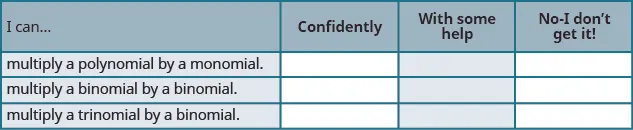What does this checklist tell you about your mastery of this section? What steps will you take to improve?

Order a print copy

As an Amazon Associate we earn from qualifying purchases.